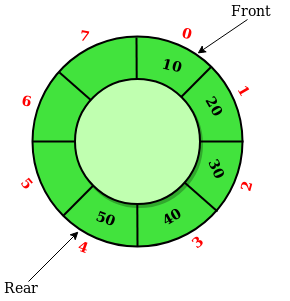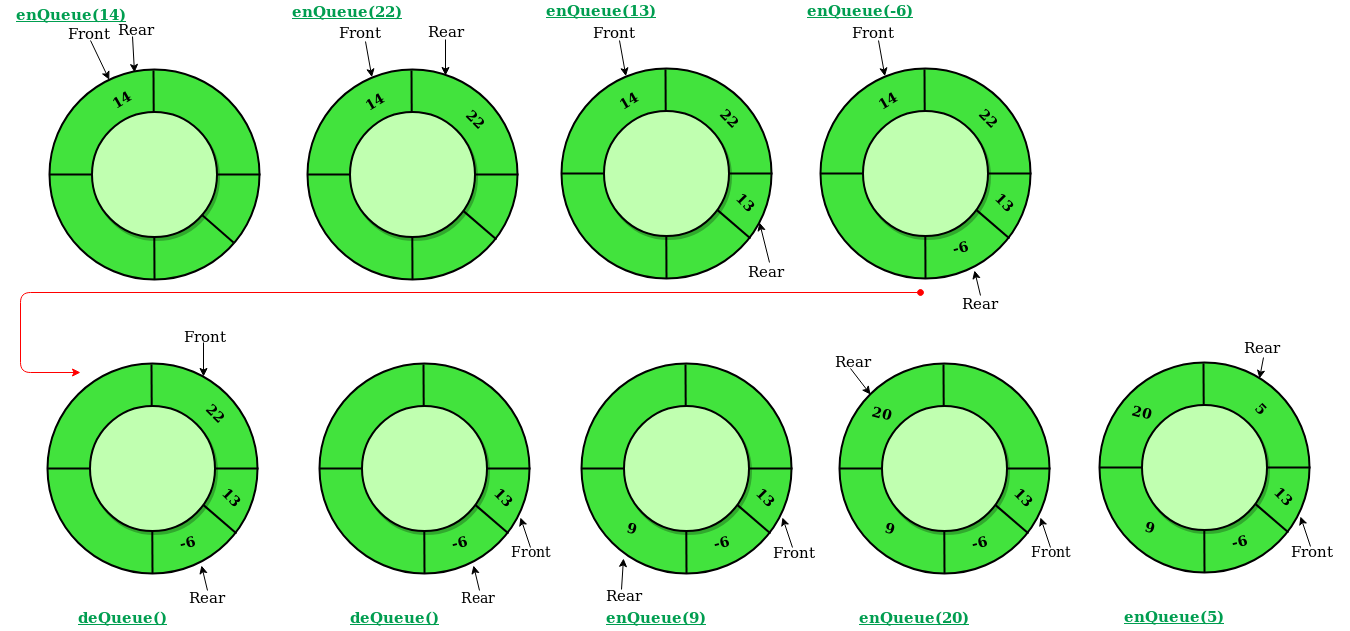# Circular Queue | Set 1 (Introduction and Array Implementation)

• Difficulty Level : Easy
• Last Updated : 10 Jun, 2022

Prerequisite – Queues
Circular Queue is a linear data structure in which the operations are performed based on FIFO (First In First Out) principle and the last position is connected back to the first position to make a circle. It is also called ‘Ring Buffer’In a normal Queue, we can insert elements until queue becomes full. But once queue becomes full, we can not insert the next element even if there is a space in front of queue.Operations on Circular Queue:

• Front: Get the front item from queue.
• Rear: Get the last item from queue.
• enQueue(value) This function is used to insert an element into the circular queue. In a circular queue, the new element is always inserted at Rear position.
1. Check whether queue is Full – Check ((rear == SIZE-1 && front == 0) || (rear == front-1)).
2. If it is full then display Queue is full. If queue is not full then, check if (rear == SIZE – 1 && front != 0) if it is true then set rear=0 and insert element.
• deQueue() This function is used to delete an element from the circular queue. In a circular queue, the element is always deleted from front position.
1. Check whether queue is Empty means check (front==-1).
2. If it is empty then display Queue is empty. If queue is not empty then step 3
3. Check if (front==rear) if it is true then set front=rear= -1 else check if (front==size-1), if it is true then set front=0 and return the element.

## C++

 `// C or C++ program for insertion and``// deletion in Circular Queue``#include``using` `namespace` `std;` `class` `Queue``{``    ``// Initialize front and rear``    ``int` `rear, front;` `    ``// Circular Queue``    ``int` `size;``    ``int` `*arr;``public``:``    ``Queue(``int` `s)``    ``{``       ``front = rear = -1;``       ``size = s;``       ``arr = ``new` `int``[s];``    ``}` `    ``void` `enQueue(``int` `value);``    ``int` `deQueue();``    ``void` `displayQueue();``};`  `/* Function to create Circular queue */``void` `Queue::enQueue(``int` `value)``{``    ``if` `((front == 0 && rear == size-1) ||``            ``(rear == (front-1)%(size-1)))``    ``{``        ``printf``(``"\nQueue is Full"``);``        ``return``;``    ``}` `    ``else` `if` `(front == -1) ``/* Insert First Element */``    ``{``        ``front = rear = 0;``        ``arr[rear] = value;``    ``}` `    ``else` `if` `(rear == size-1 && front != 0)``    ``{``        ``rear = 0;``        ``arr[rear] = value;``    ``}` `    ``else``    ``{``        ``rear++;``        ``arr[rear] = value;``    ``}``}` `// Function to delete element from Circular Queue``int` `Queue::deQueue()``{``    ``if` `(front == -1)``    ``{``        ``printf``(``"\nQueue is Empty"``);``        ``return` `INT_MIN;``    ``}` `    ``int` `data = arr[front];``    ``arr[front] = -1;``    ``if` `(front == rear)``    ``{``        ``front = -1;``        ``rear = -1;``    ``}``    ``else` `if` `(front == size-1)``        ``front = 0;``    ``else``        ``front++;` `    ``return` `data;``}` `// Function displaying the elements``// of Circular Queue``void` `Queue::displayQueue()``{``    ``if` `(front == -1)``    ``{``        ``printf``(``"\nQueue is Empty"``);``        ``return``;``    ``}``    ``printf``(``"\nElements in Circular Queue are: "``);``    ``if` `(rear >= front)``    ``{``        ``for` `(``int` `i = front; i <= rear; i++)``            ``printf``(``"%d "``,arr[i]);``    ``}``    ``else``    ``{``        ``for` `(``int` `i = front; i < size; i++)``            ``printf``(``"%d "``, arr[i]);` `        ``for` `(``int` `i = 0; i <= rear; i++)``            ``printf``(``"%d "``, arr[i]);``    ``}``}` `/* Driver of the program */``int` `main()``{``    ``Queue q(5);` `    ``// Inserting elements in Circular Queue``    ``q.enQueue(14);``    ``q.enQueue(22);``    ``q.enQueue(13);``    ``q.enQueue(-6);` `    ``// Display elements present in Circular Queue``    ``q.displayQueue();` `    ``// Deleting elements from Circular Queue``    ``printf``(``"\nDeleted value = %d"``, q.deQueue());``    ``printf``(``"\nDeleted value = %d"``, q.deQueue());` `    ``q.displayQueue();` `    ``q.enQueue(9);``    ``q.enQueue(20);``    ``q.enQueue(5);` `    ``q.displayQueue();` `    ``q.enQueue(20);``    ``return` `0;``}`

## Java

 `// Java program for insertion and``// deletion in Circular Queue``import` `java.util.ArrayList;` `class` `CircularQueue{` `// Declaring the class variables.``private` `int` `size, front, rear;` `// Declaring array list of integer type.``private` `ArrayList queue = ``new` `ArrayList();` `// Constructor``CircularQueue(``int` `size)``{``    ``this``.size = size;``    ``this``.front = ``this``.rear = -``1``;``}` `// Method to insert a new element in the queue.``public` `void` `enQueue(``int` `data)``{``    ` `    ``// Condition if queue is full.``    ``if``((front == ``0` `&& rear == size - ``1``) ||``      ``(rear == (front - ``1``) % (size - ``1``)))``    ``{``        ``System.out.print(``"Queue is Full"``);``    ``}` `    ``// condition for empty queue.``    ``else` `if``(front == -``1``)``    ``{``        ``front = ``0``;``        ``rear = ``0``;``        ``queue.add(rear, data);``    ``}` `    ``else` `if``(rear == size - ``1` `&& front != ``0``)``    ``{``        ``rear = ``0``;``        ``queue.set(rear, data);``    ``}` `    ``else``    ``{``        ``rear = (rear + ``1``);``    ` `        ``// Adding a new element if``        ``if``(front <= rear)``        ``{``            ``queue.add(rear, data);``        ``}``    ` `        ``// Else updating old value``        ``else``        ``{``            ``queue.set(rear, data);``        ``}``    ``}``}` `// Function to dequeue an element``// form th queue.``public` `int` `deQueue()``{``    ``int` `temp;` `    ``// Condition for empty queue.``    ``if``(front == -``1``)``    ``{``        ``System.out.print(``"Queue is Empty"``);``        ` `        ``// Return -1 in case of empty queue``        ``return` `-``1``;``    ``}` `    ``temp = queue.get(front);` `    ``// Condition for only one element``    ``if``(front == rear)``    ``{``        ``front = -``1``;``        ``rear = -``1``;``    ``}` `    ``else` `if``(front == size - ``1``)``    ``{``        ``front = ``0``;``    ``}``    ``else``    ``{``        ``front = front + ``1``;``    ``}``    ` `    ``// Returns the dequeued element``    ``return` `temp;``}` `// Method to display the elements of queue``public` `void` `displayQueue()``{``    ` `    ``// Condition for empty queue.``    ``if``(front == -``1``)``    ``{``        ``System.out.print(``"Queue is Empty"``);``        ``return``;``    ``}` `    ``// If rear has not crossed the max size``    ``// or queue rear is still greater then``    ``// front.``    ``System.out.print(``"Elements in the "` `+``                     ``"circular queue are: "``);` `    ``if``(rear >= front)``    ``{``    ` `        ``// Loop to print elements from``        ``// front to rear.``        ``for``(``int` `i = front; i <= rear; i++)``        ``{``            ``System.out.print(queue.get(i));``            ``System.out.print(``" "``);``        ``}``        ``System.out.println();``    ``}` `    ``// If rear crossed the max index and``    ``// indexing has started in loop``    ``else``    ``{``        ` `        ``// Loop for printing elements from``        ``// front to max size or last index``        ``for``(``int` `i = front; i < size; i++)``        ``{``            ``System.out.print(queue.get(i));``            ``System.out.print(``" "``);``        ``}` `        ``// Loop for printing elements from``        ``// 0th index till rear position``        ``for``(``int` `i = ``0``; i <= rear; i++)``        ``{``            ``System.out.print(queue.get(i));``            ``System.out.print(``" "``);``        ``}``        ``System.out.println();``    ``}``}` `// Driver code``public` `static` `void` `main(String[] args)``{``    ` `    ``// Initialising new object of``    ``// CircularQueue class.``    ``CircularQueue q = ``new` `CircularQueue(``5``);``    ` `    ``q.enQueue(``14``);``    ``q.enQueue(``22``);``    ``q.enQueue(``13``);``    ``q.enQueue(-``6``);``    ` `    ``q.displayQueue();` `    ``int` `x = q.deQueue();` `    ``// Checking for empty queue.``    ``if``(x != -``1``)``    ``{``        ``System.out.print(``"Deleted value = "``);``        ``System.out.println(x);``    ``}` `    ``x = q.deQueue();` `    ``// Checking for empty queue.``    ``if``(x != -``1``)``    ``{``        ``System.out.print(``"Deleted value = "``);``        ``System.out.println(x);``    ``}` `    ``q.displayQueue();``    ` `    ``q.enQueue(``9``);``    ``q.enQueue(``20``);``    ``q.enQueue(``5``);``    ` `    ``q.displayQueue();``    ` `    ``q.enQueue(``20``);``}``}` `// This code is contributed by Amit Mangal.`

## C#

 `// C# program for insertion and``// deletion in Circular Queue``using` `System;``using` `System.Collections.Generic;` `public` `class` `CircularQueue{``    ` `    ``// Declaring the class variables.``    ``private` `int` `size, front, rear;``     ` `    ``// Declaring array list of integer type.``    ``private` `List<``int``> queue = ``new` `List<``int``>();``    ` `    ``// Constructor``    ``CircularQueue(``int` `size)``    ``{``        ``this``.size = size;``        ``this``.front = ``this``.rear = -1;``    ``}``    ` `    ``// Method to insert a new element in the queue.``    ``public` `void` `enQueue(``int` `data)``    ``{``         ` `        ``// Condition if queue is full.``        ``if``((front == 0 && rear == size - 1) ||``          ``(rear == (front - 1) % (size - 1)))``        ``{``            ``Console.Write(``"Queue is Full"``);``        ``}``     ` `        ``// condition for empty queue.``        ``else` `if``(front == -1)``        ``{``            ``front = 0;``            ``rear = 0;``            ``queue.Add(data);``        ``}``     ` `        ``else` `if``(rear == size - 1 && front != 0)``        ``{``            ``rear = 0;``            ``queue[rear]=data;``        ``}``     ` `        ``else``        ``{``            ``rear = (rear + 1);``         ` `            ``// Adding a new element if``            ``if``(front <= rear)``            ``{``                ``queue.Add(data);``            ``}``         ` `            ``// Else updating old value``            ``else``            ``{``                ``queue[rear]=data;``            ``}``        ``}``    ``}``    ` `    ``// Function to dequeue an element``    ``// form th queue.``    ``public` `int` `deQueue()``    ``{``        ``int` `temp;``     ` `        ``// Condition for empty queue.``        ``if``(front == -1)``        ``{``            ``Console.Write(``"Queue is Empty"``);``             ` `            ``// Return -1 in case of empty queue``            ``return` `-1;``        ``}``     ` `        ``temp = queue[front];``     ` `        ``// Condition for only one element``        ``if``(front == rear)``        ``{``            ``front = -1;``            ``rear = -1;``        ``}``     ` `        ``else` `if``(front == size - 1)``        ``{``            ``front = 0;``        ``}``        ``else``        ``{``            ``front = front + 1;``        ``}``         ` `        ``// Returns the dequeued element``        ``return` `temp;``    ``}``     ` `    ``// Method to display the elements of queue``    ``public` `void` `displayQueue()``    ``{``         ` `        ``// Condition for empty queue.``        ``if``(front == -1)``        ``{``            ``Console.Write(``"Queue is Empty"``);``            ``return``;``        ``}``     ` `        ``// If rear has not crossed the max size``        ``// or queue rear is still greater then``        ``// front.``        ``Console.Write(``"Elements in the circular queue are: "``);``     ` `        ``if``(rear >= front)``        ``{``         ` `            ``// Loop to print elements from``            ``// front to rear.``            ``for``(``int` `i = front; i <= rear; i++)``            ``{``                ``Console.Write(queue[i]);``                ``Console.Write(``" "``);``            ``}``            ``Console.Write(``"\n"``);``        ``}``     ` `        ``// If rear crossed the max index and``        ``// indexing has started in loop``        ``else``        ``{``             ` `            ``// Loop for printing elements from``            ``// front to max size or last index``            ``for``(``int` `i = front; i < size; i++)``            ``{``                ``Console.Write(queue[i]);``                ``Console.Write(``" "``);``            ``}``     ` `            ``// Loop for printing elements from``            ``// 0th index till rear position``            ``for``(``int` `i = 0; i <= rear; i++)``            ``{``                ``Console.Write(queue[i]);``                ``Console.Write(``" "``);``            ``}``            ``Console.Write(``"\n"``);``        ``}``    ``}``    ` `    ``// Driver code``    ``static` `public` `void` `Main (){``        ``// Initialising new object of``        ``// CircularQueue class.``        ``CircularQueue q = ``new` `CircularQueue(5);``         ` `        ``q.enQueue(14);``        ``q.enQueue(22);``        ``q.enQueue(13);``        ``q.enQueue(-6);``         ` `        ``q.displayQueue();``     ` `        ``int` `x = q.deQueue();``     ` `        ``// Checking for empty queue.``        ``if``(x != -1)``        ``{``            ``Console.Write(``"Deleted value = "``);``            ``Console.Write(x+``"\n"``);``        ``}``     ` `        ``x = q.deQueue();``     ` `        ``// Checking for empty queue.``        ``if``(x != -1)``        ``{``            ``Console.Write(``"Deleted value = "``);``            ``Console.Write(x+``"\n"``);``        ``}``     ` `        ``q.displayQueue();``         ` `        ``q.enQueue(9);``        ``q.enQueue(20);``        ``q.enQueue(5);``         ` `        ``q.displayQueue();``         ` `        ``q.enQueue(20);``    ``}``}` `// This code is contributed by shruti456rawal`

## Python 3

 `class` `CircularQueue():` `    ``# constructor``    ``def` `__init__(``self``, size): ``# initializing the class``        ``self``.size ``=` `size``        ` `        ``# initializing queue with none``        ``self``.queue ``=` `[``None` `for` `i ``in` `range``(size)]``        ``self``.front ``=` `self``.rear ``=` `-``1` `    ``def` `enqueue(``self``, data):``        ` `        ``# condition if queue is full``        ``if` `((``self``.rear ``+` `1``) ``%` `self``.size ``=``=` `self``.front):``            ``print``(``" Queue is Full\n"``)``            ` `        ``# condition for empty queue``        ``else` `if` `(``self``.front ``=``=` `-``1``):``            ``self``.front ``=` `0``            ``self``.rear ``=` `0``            ``self``.queue[``self``.rear] ``=` `data``        ``else``:``            ` `            ``# next position of rear``            ``self``.rear ``=` `(``self``.rear ``+` `1``) ``%` `self``.size``            ``self``.queue[``self``.rear] ``=` `data``            ` `    ``def` `dequeue(``self``):``        ``if` `(``self``.front ``=``=` `-``1``): ``# condition for empty queue``            ``print` `(``"Queue is Empty\n"``)``            ` `        ``# condition for only one element``        ``else` `if` `(``self``.front ``=``=` `self``.rear):``            ``temp``=``self``.queue[``self``.front]``            ``self``.front ``=` `-``1``            ``self``.rear ``=` `-``1``            ``return` `temp``        ``else``:``            ``temp ``=` `self``.queue[``self``.front]``            ``self``.front ``=` `(``self``.front ``+` `1``) ``%` `self``.size``            ``return` `temp` `    ``def` `display(``self``):``    ` `        ``# condition for empty queue``        ``if``(``self``.front ``=``=` `-``1``):``            ``print` `(``"Queue is Empty"``)` `        ``else` `if` `(``self``.rear >``=` `self``.front):``            ``print``(``"Elements in the circular queue are:"``,``                                              ``end ``=` `" "``)``            ``for` `i ``in` `range``(``self``.front, ``self``.rear ``+` `1``):``                ``print``(``self``.queue[i], end ``=` `" "``)``            ``print` `()` `        ``else``:``            ``print` `(``"Elements in Circular Queue are:"``,``                                           ``end ``=` `" "``)``            ``for` `i ``in` `range``(``self``.front, ``self``.size):``                ``print``(``self``.queue[i], end ``=` `" "``)``            ``for` `i ``in` `range``(``0``, ``self``.rear ``+` `1``):``                ``print``(``self``.queue[i], end ``=` `" "``)``            ``print` `()` `        ``if` `((``self``.rear ``+` `1``) ``%` `self``.size ``=``=` `self``.front):``            ``print``(``"Queue is Full"``)` `# Driver Code``ob ``=` `CircularQueue(``5``)``ob.enqueue(``14``)``ob.enqueue(``22``)``ob.enqueue(``13``)``ob.enqueue(``-``6``)``ob.display()``print` `(``"Deleted value = "``, ob.dequeue())``print` `(``"Deleted value = "``, ob.dequeue())``ob.display()``ob.enqueue(``9``)``ob.enqueue(``20``)``ob.enqueue(``5``)``ob.display()` `# This code is contributed by AshwinGoel`

Output

```Elements in Circular Queue are: 14 22 13 -6
Deleted value = 14
Deleted value = 22
Elements in Circular Queue are: 13 -6
Elements in Circular Queue are: 13 -6 9 20 5
Queue is Full```

Time Complexity: Time complexity of enQueue(), deQueue() operation is O(1) as there is no loop in any of the operation.
Applications:

1. Memory Management: The unused memory locations in the case of ordinary queues can be utilized in circular queues.
2. Traffic system: In computer controlled traffic system, circular queues are used to switch on the traffic lights one by one repeatedly as per the time set.
3. CPU Scheduling: Operating systems often maintain a queue of processes that are ready to execute or that are waiting for a particular event to occur.